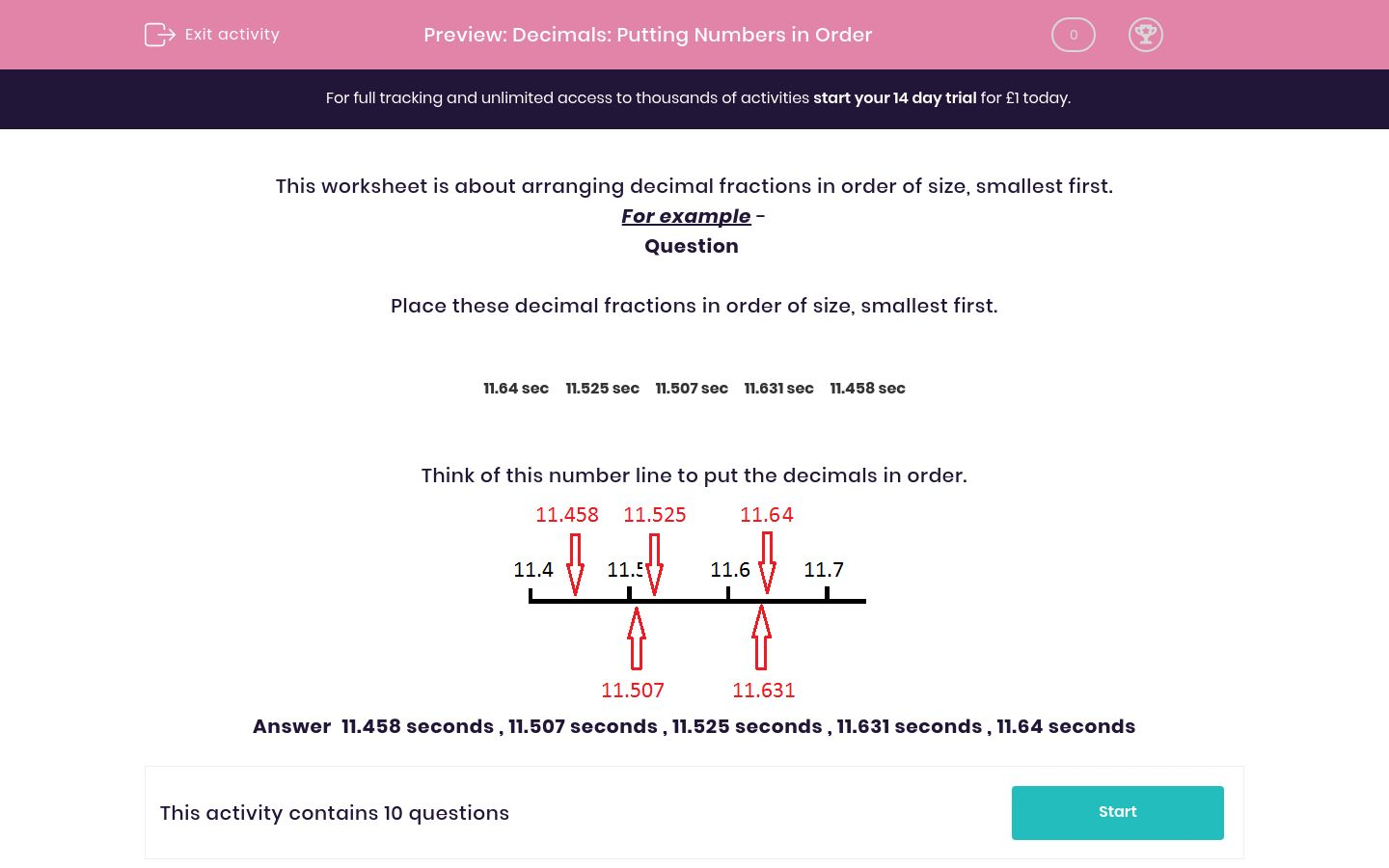# Decimals: Putting Numbers in Order

In this worksheet, students arrange decimal fractions in order of size, smallest first. A number line is used to illustrate the example.Key stage:  KS 2

Curriculum topic:   Maths and Numerical Reasoning

Curriculum subtopic:   Decimals

Difficulty level:### QUESTION 1 of 10

This worksheet is about arranging decimal fractions in order of size, smallest first.

For example -

Question

Place these decimal fractions in order of size, smallest first.

11.64 sec
11.525 sec
11.507 sec
11.631 sec
11.458 sec

Think of this number line to put the decimals in order.Answer  11.458 seconds , 11.507 seconds , 11.525 seconds , 11.631 seconds , 11.64 seconds

---- OR ----